Next: Mie Scattering Up: Multipole Expansion Previous: Radiation from Linear Centre-Fed

# Spherical Wave Expansion of Vector Plane Wave

In discussing the scattering or absorption of electromagnetic radiation by localized systems, it is useful to be able to express a plane electromagnetic wave as a superposition of spherical waves.

Consider, first of all, the expansion of a scalar plane wave as a set of scalar spherical waves. This expansion is conveniently obtained from the expansion (1517) for the Green's function of the scalar Helmholtz equation. Let us take the limit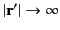of this equation. We can make the substitution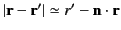on the left-hand-side, where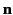is a unit vector pointing in the direction of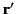. On the right-hand side,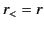and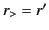. Furthermore, we can use the asymptotic form (1432) for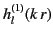. Thus, we obtain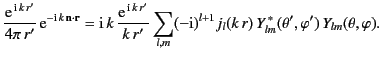(1566)

Canceling the factor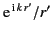on either side of the above equation, and taking the complex conjugate, we get the following expansion for a scalar plane wave,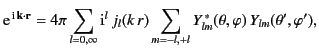(1567)

where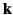is a wavevector with the spherical coordinates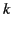,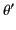,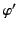. The well-known addition theorem for the spherical harmonics states that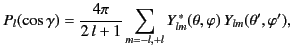(1568)

where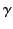is the angle subtended between the vectors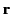and. Consequently,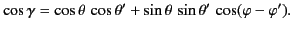(1569)

It follows from Equations (1569) and (1570) that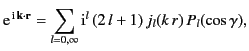(1570)

or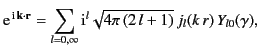(1571)

because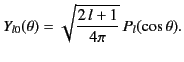(1572)

Let us now make an equivalent expansion for a circularly polarized plane wave incident along the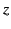-axis: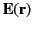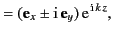(1573)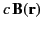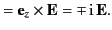(1574)

Because the plane wave is finite everywhere (including the origin), its multipole expansion (1479)-(1480) can only involve the well-behaved radial eigenfunctions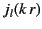. Thus,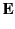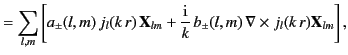(1575)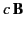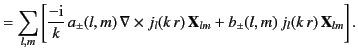(1576)

To determine the coefficients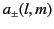and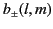, we make use of a slight generalization of the standard orthogonality properties (1477)-(1478) of the vector spherical harmonics: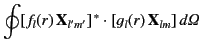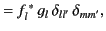(1577)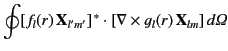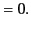(1578)

The first of these follows directly from Equation (1477). The second follows from Equations (1442), (1478), (1486), and the identity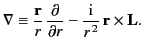(1579)

The coefficientsandare obtained by taking the scalar product of Equations (1577)-(1578) with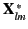and integrating over all solid angle, making use of the orthogonality relations (1579)-(1580). This yields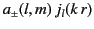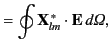(1580)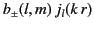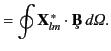(1581)

Substitution of Equations (1476) and (1577) into Equation (1582) gives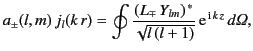(1582)

where the operators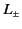are defined in Equations (1439)-(1440). Making use of Equations (1444)-(1446), the above expression reduces to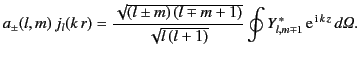(1583)

If the expansion (1573) is substituted for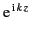, and use is made of the orthogonality properties of the spherical harmonics, then we obtain the result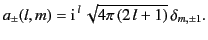(1584)

It is clear from Equations (1576) and (1583) that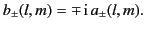(1585)

Thus, the general expansion of a circularly polarized plane wave takes the form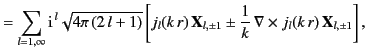(1586)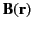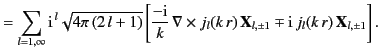(1587)

The expansion for a linearly polarized plane wave is easily obtained by taking the appropriate linear combination of the above two expansions.Next: Mie Scattering Up: Multipole Expansion Previous: Radiation from Linear Centre-Fed
Richard Fitzpatrick 2014-06-27